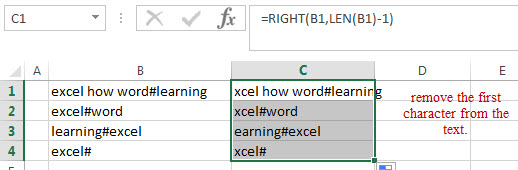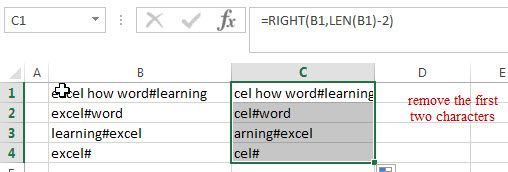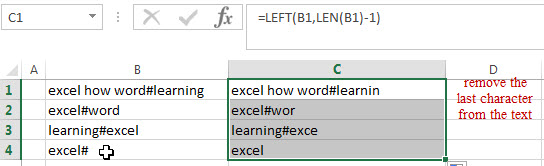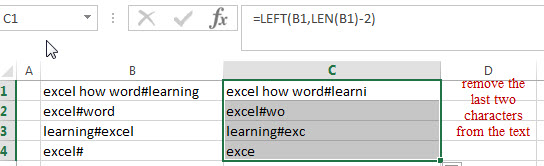# How to remove first and last characters from text string

This post will guide you how to remove the first character from a text string using excel formula. How to remove the last character from string in Excel. How to remove the first x number of characters from a text string. How to delete the last x number of characters from string.

## Remove First Character

If you want to remove the first character from the beginning of the text string, you can use a combination of the RIGHT function and the LEN function to create an excel formula.

You can use the RIGHT function to extract the rightmost characters and the length of the character that you want to extract can get by the LEN function, the number is then subtracted 1 to get the length of the characters that you want to extract. Assuming that you want to remove the first character from the text string in Cell B1, then you can write down the following formula:

`=RIGHT(B1,LEN(B1)-1)`This formula will extract the right-most characters from the text in Cell B1 and the length of the characters that you need to extract is returned by the LEN(B1)-1 formula, so it will remove the first character from the text.

## Remove First Two Character

If you want to remove the first two characters, then you just need to use the number 2 instead of 1 for the above formula. So you can use the following formula:

`=RIGHT(B1,LEN(B1)-2)`## Remove Last character

If you want to remove the last character from the text string in Cell B1, you can use the LEN function to combine with the LEFT function to create an excel formula.

You can use the LEFT function to extract the left-most number of characters, and the length of the characters that you want to extract is returned by the formula LEN(B1)-1. It is also the length of the characters after the last character is removed. So you can write down the below formula:

`=LEFT(B1,LEN(B1)-1)`This formula will extract the left-most number of characters, the LEN function returns the length of the original text and then is subtracted to 1 to get the length of the characters that you want to extract.  Then it will remove the last character from the text.

## Remove Last two Characters

If you want to remove the last two characters from the text in Cell B1, you can use the following formula:

`=LEFT(B1,LEN(B1)-2)`### Related Formulas

• Remove unwanted characters from text string
If you want to remove unwanted or specified characters from a text string, you can create an excel formula based on the SUBSTITUTE function and CHAR function….
• Extract Text between Parentheses
If you want to extract text between parentheses in a cell, then you can use the search function within the MID function to create a new excel formula…
• Remove text before the first match of a specific character
If you want to remove all characters that before the first occurrence of the comma character, you can use a formula based on the RIGHT function, the LEN function and the FIND function…..
• Extract Text between Brackets
If you want to extract text between brackets in a cell, you need to create a formula based on the SEARCH function and the MID function….
• Extract Text between Commas
To extract text between commas in Cell B1, you can use the following formula based on the SUBSTITUTE function, the MID function and the REPT function…..
• Extract word that starting with a specific character
Assuming that you have a text string that contains email address in Cell B1, and if you want to extract word that begins with a specific character “@” sign, you can use a combination with the TRIM function, the LEFT function, the SUBSTITUTE function ….
• Extract text before first comma or space
If you want to extract text before the first comma or space character in cell B1, you can use a combination of the LEFT function and FIND function….
• Extract text after first comma or space
If you want to get substring after the first comma character from a text string in Cell B1, then you can create a formula based on the MID function and FIND function or SEARCH function ….
• Extract text before the second or nth specific character
you can create a formula based on the LEFT function, the FIND function and the SUBSTITUTE function to Extract text before the second or nth specific character…

### Related Functions

• Excel LEFT function
The Excel LEFT function returns a substring (a specified number of the characters) from a text string, starting from the leftmost character.The LEFT function is a build-in function in Microsoft Excel and it is categorized as a Text Function.The syntax of the LEFT function is as below:= LEFT(text,[num_chars])…t)…
• Excel RIGHT function
The Excel RIGHT function returns a substring (a specified number of the characters) from a text string, starting from the rightmost character.The syntax of the RIGHT function is as below:= RIGHT (text,[num_chars])…
• Excel LEN function
The Excel LEN function returns the length of a text string (the number of characters in a text string).The syntax of the LEN function is as below:= LEN(text)…